PRINTABLE FOR KIDS

XII (12) HSC

XI (11) FYJC
X (10) SSC

### Sets Class 9th Mathematics Part I MHB Solution

##### Sets Class 9th Mathematics Part I MHB Solution

###### Practice Set 1.1
Question 1.

Write the following sets in roster form.
i. Set of even numbers
ii. Set of even prime numbers from 1 to 50
iii. Set of negative integers
iv. Seven basic sounds of a sargam (sur)

We know that in roster method, we write all the elements of a set in curly bracket. Each of the element is written only once and separated by commas. The order of an element is not important but it is necessary to write all the elements of the set.

i. A = {2, 4, 6, 8, 10, 12, 14, … }

ii. B = {2}

iii. C = { … -7, -6, -5, -4, -3, -2, -1}

iv. D = {Sa, Re, Ga, Ma, Pa, Dha, Ni}

Question 2.

Write the following symbolic statements in words.

i. 4/3 ∈ Q

ii. - 2 N

iii. P = {P is an odd number}

i. We know that Q is denoted as set of rational numbers.

We write 4/3 ∈ Q as ‘4/3 is an element of set of rational numbers’.

ii. We know that N is denoted as set of natural numbers.

We write -2 N as ‘-2 is not an element of set of natural numbers.’

iii. We write P = {p| p is an odd number} as ‘Set P contains all the odd numbers’.

Question 3.

Write any two sets by listing method and by rule method.

We know that in listing method, we write all the elements of a set in curly bracket. Each of the elements is written only once and separated by commas. The order of an element is not important but it is necessary to write all the elements of the set and In rule method, we do not write the list of elements but write the general element using variable followed by a vertical line or colon and write the property of the variable.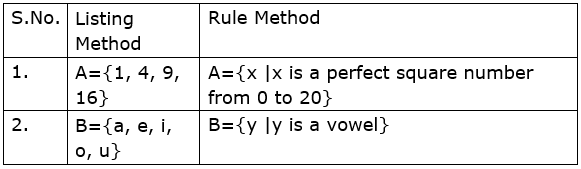Question 4.

Write the following sets using listing method.

i. All months in the Indian solar year.

ii. Letters in the word ‘COMPLEMENT’.

iii. Set of human sensory organs.

iv. Set of prime numbers from 1 to 20.

v. Names of continents of the world.

i. We know that in listing method, we write all the elements of a set in curly bracket. Each of the elements is written only once and separated by commas. The order of an element is not important but it is necessary to write all the elements of the set.

A = {Mesa, Vrsabha, Mithuna, Karkata, Simha, Kanya, Tula, Vrscika, Dhanu, Makara, Kumbha, Mina}

ii. B = {C, O, M, P, L, E, N, T}

iii. C = {Eyes, Ears, Nose, Mouth, Skin}

iv. D = {2, 3, 5, 7, 11, 13, 17, 19}

v. E = {Asia, Africa, North America, South America, Antarctica, Europe, Australia}

Question 5.

Write the following sets using rule method.
i. A = {1,4,9,16,25,36,49,64,81,100}

ii. B = {6,12,18,24,30,36,42,48}

iii. C = {S, M, I, L, E}

iv. D = {Sunday, Monday, Tuesday, Wednesday, Thursday, Friday, Saturday}

We know that in rule method, we do not write the list of elements but write the general element using variable followed by a vertical line or colon and write the property of the variable.

i. A = {x| x is a perfect square number from 1 to 101}

ii. B = {y| y is a multiple of 6 and is less than 50}

iii. C = {z| z is a letter of the world ‘SMILE’.}

iv. D = {p | p is a day of the week}

###### Practice Set 1.2
Question 1.

Decide which of the following are equal sets and which are not? Justify your answer.

A = {x|3x – 1 = 2}

B = {x | x is a natural number but x is neither prime nor composite}

C = {x |x ∈ N, x < 2}

We know that two sets A and B are said to be equal, if every element of set A is in set B and every element of set B is in set A. It is symbolically written as A = B.

A = {x|3x – 1 = 2}

⇒ 3x – 1 = 2

⇒ 3x = 2 + 1 = 3

⇒ x = 3/3 = 1

∴ A = {1}

B = {x |x is a natural number but x is neither prime nor composite}

We know that 1 is neither prime nor composite and is a natural number.

∴ B = {1}

C = {x |x ∈ N, x < 2}

We know that natural number, N = {0, 1, 2, 3, …}

∴ C = {0, 1}

A = B, B ≠ C, A ≠ C

Ans. A and B are equal sets but B and C (and) A and C are not equal sets.

Question 2.

Decide whether set A and B are equal sets. Give reason for your answer.

A = Even prime numbers

B = {x| 7x – 1 = 13}

We know that two sets A and B are said to be equal, if every element of set A is in set B and every element of set B is in set A. It is symbolically written as A = B.

A = Even Prime Numbers = {2}

B = {x| 7x – 1 = 13}

⇒ 7x – 1 = 13

⇒ 7x = 13 + 1 = 14

⇒ x = 14/7

∴ x = 2

∴ A = B

Ans. Set A and set B are equal sets.

Question 3.

Which of the following are empty sets? Why?

i. A = {a |a is a natural number smaller than zero.}

ii. B = {x|x2 = 0}

iii. C = {x|5x – 2 = 0, x ∈ N}

i. We know that If there is not a single element in the set which satisfies the given condition then it is called a Null set or an Empty set. Empty set is represented by { }.

We know that Natural number, N = {0, 1, 2, … }.

∴ A = {a |a is a natural number smaller than zero} = {}

Ans. A is an empty set.

ii. B = {x|x2 = 0}

⇒ x2 = 0

∴ x = 0

B = {x|x2 = 0} = {0} ≠ {}

Ans. B is not an empty set.

iii. C = {x|5x – 2 = 0, x ∈ N}

⇒ 5x – 2 = 0

⇒ 5x = 2

⇒ x = 5/2

We know that natural number, N = {0, 1, 2,… }

∴ x N

∴ C = {x|5x – 2 = 0, x ∈ N} = {}

Ans. C is an empty set.

Question 4.

Write with reasons, which of the following sets are finite or infinite. We know that if a set is a null set or number of elements are limited and countable then it is called ‘Finite set’ and if number of elements in a set is unlimited and uncountable then the set is called ‘Infinite set’.

i. A = {x|x < 10, x is a natural number}

ii. B = {y |y< -1, is an integer}

iii. C = Set of students of class 9 from your school.

iv. Set of people from your village.

v. Set of apparatus in laboratory

vi. Set of whole numbers

vii. Set of rational number

i. A = {x|x < 10, x is a natural number}

∴ A = {0, 1, 2, 3, 4, 5, 6, 7, 8, 9}

Ans. A is a finite set.

ii. B = {y |y< -1, y is an integer}

∴ B = {… -5, -4, -3, -2}

Ans. B is an infinite set.

iii. C = {a, b, c, d, e, …}

Ans. C is an infinite set.

iv. D = {Sarpanch, villager1, villager2, …}

Ans. D is an infinite set.

v. E = {Beakers, funnels, chemicals, thermometers, …}

Ans. E is an infinite set.

vi. W = {1, 2, 3, 4, 5, …}

Ans. W is an infinite set.

vii. Q = {(p/q) |p, q ∈ I, q ≠ 0}

Q = {1/2, 1/3, 1/4, …}

Ans. Q is an infinite set.

###### Practice Set 1.3
Question 1.

If A = {a, b, c, d, e}, B = {c, d, e, f}, C = {b, d} and D = {a, e}.

then which of the following statements are true and which are false?

i. C ⊆ B ii. A ⊆ D
iii. D ⊆ B iv. D ⊆ A

v. B ⊆ A vi. C ⊆ A

We consider the following Venn diagram to check whether the above statements are true or not.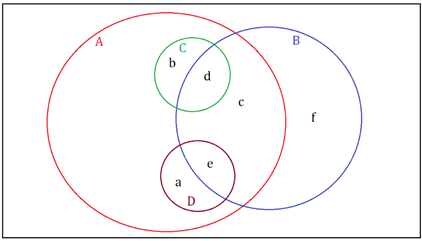Now, we have A = {a, b, c, d, e}, B = {c, d, e, f}, C = {b, d} and D = {a, e}.

So, it is clear from above that every element of set C and set D is also an element of set A.

∴ C ⊆ A and D ⊆ A

Hence, (iv), (vi) are true and (ii) is false.

Also, it is clear from above that every element of set B is not an element of set A.

∴ (v) is false.

Similarly, it is clear that every element of set C is not an element of set B.

∴ (i) is false.

Also, every element of set D is not an element of set B.

∴ (iii) is false.

Question 2.

Take the set of natural numbers from 1 to 20 as universal set and show set X and Y using Venn diagram.

i. X = {x | x ∈ ℕ, and 7 < x < 15}

ii. Y = {y | y ∈ ℕ, y is prime number from 1 to 20}

Consider the universal set:

U = {u | u ∈ ℕ, 1 ≤ x ≤ 20}

= {1, 2, 3, 4, 5, 6, 7, 8, 9, 10, 11, 12, 13, 14, 15, 16, 17, 18, 19, 20}

X = {x | x ∈ ℕ, 7 < x < 15}

= {8, 9, 10, 11, 12, 13, 14}

Y = {y | y ∈ ℕ, y is prime number from 1 to 20}

= {2, 3, 5, 7, 11, 13, 17, 19}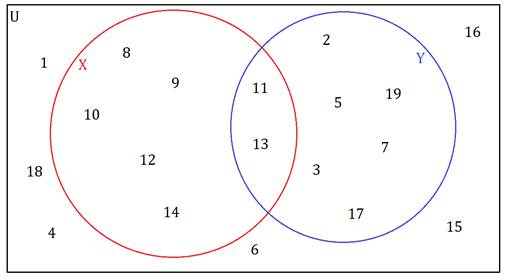Question 3.

U = {1, 2, 3, 7, 8, 9, 10, 11, 12}

P = {1, 3, 7, 10}

then (i) show the sets U, P and P � by Venn diagram. (ii) Verify (P’)’ = P

U = {1, 2, 3, 7, 8, 9, 10, 11, 12}

P = {1, 3, 7, 10}

P’ = {x | x ∈ U and x ∉ P} = {2, 8, 9, 11, 12}

(i) The Venn diagram showing U, P and P’ is: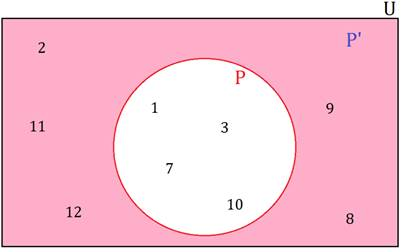(ii) Now, we consider (P’)’

(P’)’ = {x | x ∈ U and x ∉ P’}

= {1, 3, 7, 10}

= P

Hence, (P’)’ = P.

Question 4.

A = {1, 3, 2, 7} then write any three subsets of A.

We know that any set S such that every element of S is an element of the set A is said to be a subset of A.

∴ Consider B = {1, 2, 3}, C = {1, 3} and D = {2, 3, 7}

It is clear from above that every element of the sets B, C and D is an element of set A, so, B, C and D are subsets of A.

Also, note that

Every set is a subset of itself, i. e. A ⊆ A and

Empty set is a subset of every set, i. e. ϕ ⊆ A.

So, A and ϕ are also subsets of A.

Question 5.

(i) Write the subset relation between the sets.

P is the set of all residents in Pune.

M is the set of all residents in Madhya Pradesh.

I is the set of all residents in Indore.

B is the set of all residents in India.

H is the set of all residents in Maharashtra.

(ii) Which set can be the universal set for above sets?

(i) Consider the following Venn diagram for the above sets: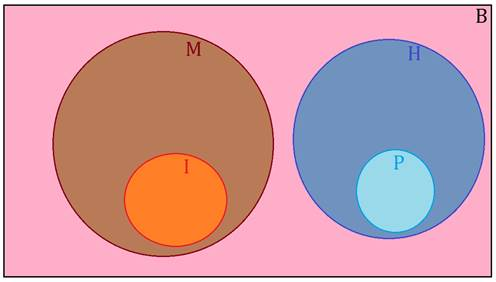(ii) From the above Venn diagram, it is clear that set B can serve as the universal set for above sets.

Question 6.

Which set of numbers could be the universal set for the sets given below?

i. A = set of multiples of 5,

B = set of multiples of 7.

C = set of multiples of 12

A universal set is the set which can accommodate all other sets in it, i.e. every element of all the sets should be an element of the universal set.

A = set of multiples of 5,

B = set of multiples of 7.

C = set of multiples of 12

Consider the set of all integers, I = {………, -2, -1, 0, 1, 2,………}

We see that every element of the sets A, B and C is an element of the set I.

So, set I can serve as the universal set for the sets A, B and C.

Question 7.

P = set of integers which multiples of are 4.

T = set of all even square numbers.

A universal set is the set which can accommodate all other sets in it, i.e. every element of all the sets should be an element of the universal set.

P = set of integers which are multiples of 4

= {x | x ∈ ℤ and x is a multiple of 4}

T = {y | y is an even square number}

Consider the set of all integers, I = {………, -2, -1, 0, 1, 2,………}

We see that every element of both the sets P and T is an element of the set I.

So, set I can serve as the universal set for the sets P and T.

Question 8.

Let all the students of a class is a Universal set. Let set A be the students who secure 50% or more marks in Maths. Then write the complement of set A.

Let U be the universal set.

Then, U = Set of all students of a class

A = Set of students who secure 50% or more marks in Maths

We know that a set A’ is said to be the complement of the set A if it contains all the elements of the universal set U, which are not in the set A.

∴The complement of set A is:

A’ = Set of all students who do not secure 50% or more marks in Maths

Or A’ = Set of all students who secured less than 50% marks in Maths
∴ A'= U - A

###### Practice Set 1.4
Question 1.

If n(A) = 15, n(A ∪ B) = 29, n (A ∩ B) = 7 then n (B) = ?

Given: n(A ∪ B) = 29, n(A) = 15 and n (A ∩ B) = 7

As we know, n (A ∪ B) = n(A) + n(B) - n (A ∩ B)

⇒ 7 = 15 + n(B) – 29

⇒ n(B) = -14 - 7

⇒ n(B) = -21

Question 2.

In a hostel there are 125 students, out of which 80 drink tea, 60 drink coffee and 20drink tea and coffee both. Find the number of students who do not drink tea or coffee.

Given: n(A) = 80, n(B) = 60 and n (A ∩ B) = x = 20

Total number of students = 125

n(A) = number of students who drink tea

n(B) = number of students who drink coffee

n (A ∩ B) = number of students who drink both tea and coffee

n (A ∪ B) = number of students who drink either tea or coffee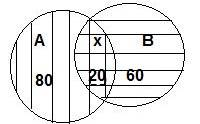As we know, n (A ∪ B) = n(A) + n(B) - n (A ∩ B)

⇒ n (A ∪ B) = 80 + 60 - 20

⇒ n (A ∪ B) = 140 - 20

⇒ n (A ∪ B) = 120

Then, the number of students who do not drink tea or coffee = 125 – 120

= 5

Question 3.

In competitive exam 50 students passed in English. 60 students passed in Mathematics.40 students passed in both the subjects. None of them fail in both the subjects. Find the number of students who passed at least in one of the subjects?

Given: n(A) = 50, n(B) = 60 and n (A ∩ B) = x = 40

n(A) = number of students who passed in English

n(B) = number of students who passed in mathematics

n (A ∩ B) = number of students who passed both subjects

n (A ∪ B) = number of students who passed in at least one of the subject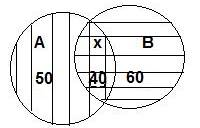As we know, n (A ∪ B) = n(A) + n(B) - n (A ∩ B)

⇒ n (A ∪ B) = 50 + 60 - 40

⇒ n (A ∪ B) = 110 - 40

⇒ n (A ∪ B) = 70

Hence, the number of students who passed in at least one of the subject = 70

Question 4.

A survey was conducted to know the hobby of 220 students of class IX. Out of which130 students informed about their hobby as rock climbing and 180 students informed about their hobby as sky watching. There are 110 students who follow both the hobbies. Then how many students do not have any of the two hobbies? How many of them follow the hobby of rock climbing only? How many students follow the hobby of sky watching only?

Given: n(A) = 130, n(B) = 180 and n (A ∩ B) = x = 110

Total number of students = 220

n(A) = number of students whose hobby is rock climbing

n(B) = number of students whose hobby is sky watching

n (A ∩ B) = number of students whose hobby is both

n (A ∪ B) = number of students whose hobby is either rock climbing or sky watching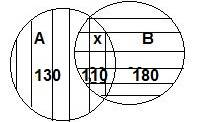As we know, n (A ∪ B) = n(A) + n(B) - n (A ∩ B)

⇒ n (A ∪ B) = 130 + 180 - 110

⇒ n (A ∪ B) = 310 - 110

⇒ n (A ∪ B) = 200

Then,

(i) The number of students who do not have any of the two hobbies

= 220– 200

= 20

(ii) The number of students whose hobby is rock climbing

= 130– 110

= 20

(iii) The number of students whose hobby is sky watching

= 180– 110

= 70

Question 5.

Observe the given Venn diagram and write the following sets.

i. A ii. B

iii. A ∪ B iv. U

C. A’ vi. B’

vii. (A ∪ B)’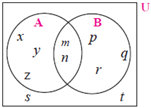(i) A = {x, y, z, m, n}

(ii) B = {p, q, r, m, n}

(iii) (A ∪ B) = { x, y, z, m, n, p, q, r}

(iv) U = { x, y, z, m, n, p, q, r, s, t}

(v) A’ = {p, q, r, s, t}

(vi) B’ = {x, y, z, s, t}

(vii) (A ∪ B)’ = { s, t}

###### Problem Set 1
Question 1.

Choose the correct alternative answer for each of the following questions.

If M = {1, 3, 5}, N = {2, 4, 6}, then M ∩ N =?
A. {1, 2, 3, 4, 5, 6}

B. {1, 3, 5}

C. ϕ

D. {2, 4, 6}

Given: M = {1, 3, 5}, N = {2, 4, 6}

(M ∩ N) = set of all common elements of M and N

Hence, (M ∩ N) = ϕ

Option (C) is correct.

Given : P = {x | x is an odd natural number,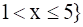set in roster form:

⇒ P = {3, 5}

Option (D) is correct.

Question 3.

Choose the correct alternative answer for each of the following questions.

P = {1, 2, ........., 10}, What type of set P is ?
A. Null set

B. Infinite set

C. Finite set

D. None of these

Given : P = {1, 2, ........., 10}

As the number of elements given are finite.

⇒ given set is finite set.

Option (C) is correct.

Question 4.

Choose the correct alternative answer for each of the following questions.

M ∪ N= {1, 2, 3, 4, 5, 6} and M = {1, 2, 4} then which of the following represent set N?
A. {1, 2, 3}

B. {3, 4, 5, 6}

C. {2, 5, 6}

D. {4, 5, 6}

Given : (M ∪ N) = {1, 2, 3, 4, 5, 6}, M = {1, 2, 4}

(M ∪ N) = set of all elements of set M and N

Hence, N = {3, 4, 5, 6}

Option (B) is correct.

Question 5.

Choose the correct alternative answer for each of the following questions.

If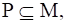then Which of the following set represent P ∩(P ∪ M)?
A. P

B. M

C. P ∪ M

D. P ∩ M

Given :As,Then using property,

(P ∪ M) = M

As,Then using property,

(P ∩ M) = P

Question 6.

Choose the correct alternative answer for each of the following questions.

Which of the following sets are empty sets?
A. set of intersecting points of parallel lines

B. set of even prime numbers.

C. Month of an english calendar having less than 30 days.

D.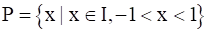A. set of intersecting points of parallel lines = ϕ

Because no two parallel lines will intersect.

Hence, Option (A) is correct.

Question 7.

Find the correct option for the given question.

Which of the following collections is a set?
A. Colours of the rainbow

B. Tall trees in the school campus.

C. Rich people in the village

D. Easy examples in the book

SET: If we can definitely and clearly decide the objects of a given collection

then that collection is called set.

Hence, option A is correct answer.

Because we can clearly say that colours of rainbow are seven in number as

(violet, indigo, blue, green, yellow, orange and red).

Hence, Option (A) is correct.

Question 8.

Find the correct option for the given question.

Which of the following set represent N ∩ W?
A. {1, 2, 3, .....}

B. {0, 1, 2, 3, ....}

C. {0}

(D) { }

Given : N = {1, 2, .........∞} and W = {0, 1, 2, .........∞}

(N ∩ W) = set of all common elements of N and W

Hence, (N ∩ W) = {1, 2, .........∞}

Option (A) is correct.

Question 9.

Find the correct option for the given question.

P = {x | x is a letter of the word ' Indian'} then which one of the following is set P in listing form?
A. {i, n, d}

B. {i, n, d, a}

C. {i,n,d,i,a}

D. {n, d, a}

Given : P = {x | x is a letter of the word ' indian'}

listing form = each of the element is written only once and separated by commas in curly brackets.

Hence, P = {i, n, d, a}

Option (B) is correct.

Question 10.

Find the correct option for the given question.

If T = {1, 2, 3, 4, 5} and M = {3, 4, 7, 8} then T ∪ M=?
A. {1, 2, 3, 4, 5, 7}

B. {1, 2, 3, 7, 8}

C. {1, 2, 3, 4, 5, 7, 8}

D. {3, 4}

Given : T = {1, 2, 3, 4, 5} and M = {3, 4, 7, 8}

(T ∪ M) = set of all elements of set T and M

Hence, (T ∪ M) = {1, 2, 3, 4, 5, 7, 8}

Option (C) is correct.

Question 11.

Out of 100 persons in a group, 72 persons speak English and 43 persons speak French. Each one out of 100 persons speak at least one language. Then how many speak only English? How many speak only French? How many of them speak English and French both?

Given: n(A) = 72, n(B) = 43 and n (A ∪ B) = 100

Total number of persons who speak at least one language = n (A ∪ B) = 100

n(A) = number of students who speaks English

n(B) = number of students who speaks French

n (A ∩ B) = number of students who speak both

As we know, n (A ∪ B) = n(A) + n(B) - n (A ∩ B)

⇒ n (A ∩ B) = 72 + 43 - 100

⇒ n (A ∩ B) = 115 - 100

⇒ n (A ∩ B) = 15

number of students who speak both the language = 15

number of students who speaks English only = 72 – 15 = 57

number of students who speaks French only = 43 – 15 = 28

Question 12.

70 trees were planted by Parth and 90 trees were planted by Pradnya on the occasion ofTree Plantation Week. Out of these; 25 trees were planted by both of them together. Howmany trees were planted by Parth or Pradnya?

Given: n(A) = 70, n(B) = 90 and n (A ∩ B) = x = 25

n(A) = number of trees planted by Parth

n(B) = number of trees planted by Pradnya

n (A ∩ B) = number of trees planted by both of them together

n (A ∪ B) = number of trees planted by Parth or Pradnya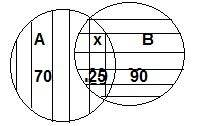As we know, n (A ∪ B) = n(A) + n(B) - n (A ∩ B)

⇒ n (A ∪ B) = 70 + 90 - 25

⇒ n (A ∪ B) = 160 - 25

⇒ n (A ∪ B) = 35

Question 13.

If n(A) = 20, n(B) = 28 and n (A ∪ B) = 36 then n (A ∩ B) = ?

Given: n(A) = 20, n(B) = 28 and n (A ∪ B) = 36

As we know, n (A ∪ B) = n(A) + n(B) - n (A ∩ B)

⇒ n (A ∩ B) = 20 + 28 - 36

⇒ n (A ∩ B) = 48 - 36

⇒ n (A ∩ B)) = 12

Question 14.

In a class, 8 students out of 28 have a dog as their pet animal at home, 6 students have a cat as their pet animal. 10 students have dog and cat both, then how many students do not have a dog or cat as their pet animal at home?

Given: n(A) = 8, n(B) = 6 and n (A ∩ B) = 10

n(A) = number of students having only dog as pet

n(B) = number of students having only cat as pet

n (A ∩ B) = number of trees planted by both of them together

Total students having a dog or cat as their pet animal at home = 8 + 6 + 10

= 24

Hence, number of students do not have a dog or cat as their pet animal at

Home = 28 – 24 = 4

Question 15.

Represent the union of two sets by Venn diagram for each of the following.

A = {3, 4, 5, 7}

B = {1, 4, 8}

Given: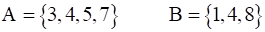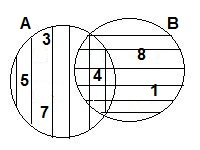Question 16.

Represent the union of two sets by Venn diagram for each of the following.

P = {a, b, c, e, f}

Q = {I, m, n, e, b}

Given: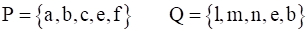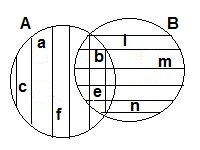Question 17.

Represent the union of two sets by Venn diagram for each of the following.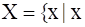is a prime number between 80 and 100}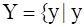is an odd number between 90 and 100}

Given: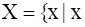is a prime number between 80 and 100}is an odd number between 90 and 100}

X = {83, 89, 93, 97}

Y = {91, 93, 95, 97, 99}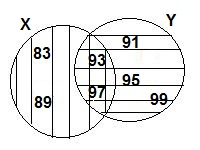Question 18.

Write the subset relations between the following sets.

X = set of all quadrilaterals.

Y = set of all rhombuses.

S = set of all squares.

T = set of all parallelograms.

V = set of all rectangles.

Given: X = set of all quadrilaterals.

Y = set of all rhombuses.

S = set of all squares.

T = set of all parallelograms.

V = set of all rectangles.

S ⊆ X, because all squares are quadrilaterals.

V ⊆ X, because all rectangles are quadrilaterals.

T ⊆ X, because all parallelograms are quadrilaterals.

S ⊆ Y, because all squares are rhombus.

S ⊆ V, because all squares are rectangles.

S ⊆ T, because all squares are parallelograms.

V ⊆ T, because all rectangles are parallelograms.

Y ⊆ T, because all rhombus are parallelograms.

Question 19.

If M is any set, then write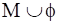and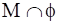Given: M is any set

As we know ϕ is null set.

Hence,

(M ∪ ϕ ) = M

(M ∩ ϕ ) = ϕ

Question 20.

Observe the Venn diagram and write the given sets U,A,B,A ∪ B and A ∩ B.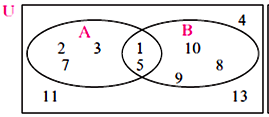(i) U = {1, 2, 3, 4, 5, 7, 8, 9, 10, 11, 13}

(ii) A = {1, 2, 3, 5, 7}

(iii) B = {1, 5, 8, 9, 10}

(iv) (A ∪ B ) = {1, 2, 3, 5, 7, 8, 9, 10}

(v) (A ∩ B ) = {1, 5}

Question 21.

If n(A) = 7, n(B) = 13, n (A ∩ B) =4 then n (A ∪ B) = ?

Given: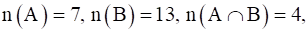As we know, n (A ∪ B) = n(A) + n(B) - n (A ∩ B)

⇒ n (A ∪ B) = 7 + 13 - 4

⇒ n (A ∪ B) = 20 - 4

⇒ n (A ∪ B) = 16

## PDF FILE TO YOUR EMAIL IMMEDIATELY PURCHASE NOTES & PAPER SOLUTION. @ Rs. 50/- each (GST extra)

SUBJECTS

HINDI ENTIRE PAPER SOLUTION

MARATHI PAPER SOLUTION
SSC MATHS I PAPER SOLUTION
SSC MATHS II PAPER SOLUTION
SSC SCIENCE I PAPER SOLUTION
SSC SCIENCE II PAPER SOLUTION
SSC ENGLISH PAPER SOLUTION
SSC & HSC ENGLISH WRITING SKILL
HSC ACCOUNTS NOTES
HSC OCM NOTES
HSC ECONOMICS NOTES
HSC SECRETARIAL PRACTICE NOTES

2019 Board Paper Solution

HSC ENGLISH SET A 2019 21st February, 2019

HSC ENGLISH SET B 2019 21st February, 2019

HSC ENGLISH SET C 2019 21st February, 2019

HSC ENGLISH SET D 2019 21st February, 2019

SECRETARIAL PRACTICE (S.P) 2019 25th February, 2019

HSC XII PHYSICS 2019 25th February, 2019

CHEMISTRY XII HSC SOLUTION 27th, February, 2019

OCM PAPER SOLUTION 2019 27th, February, 2019

HSC MATHS PAPER SOLUTION COMMERCE, 2nd March, 2019

HSC MATHS PAPER SOLUTION SCIENCE 2nd, March, 2019

SSC ENGLISH STD 10 5TH MARCH, 2019.

HSC XII ACCOUNTS 2019 6th March, 2019

HSC XII BIOLOGY 2019 6TH March, 2019

HSC XII ECONOMICS 9Th March 2019

SSC Maths I March 2019 Solution 10th Standard11th, March, 2019

SSC MATHS II MARCH 2019 SOLUTION 10TH STD.13th March, 2019

SSC SCIENCE I MARCH 2019 SOLUTION 10TH STD. 15th March, 2019.

SSC SCIENCE II MARCH 2019 SOLUTION 10TH STD. 18th March, 2019.

SSC SOCIAL SCIENCE I MARCH 2019 SOLUTION20th March, 2019

SSC SOCIAL SCIENCE II MARCH 2019 SOLUTION, 22nd March, 2019

XII CBSE - BOARD - MARCH - 2019 ENGLISH - QP + SOLUTIONS, 2nd March, 2019

HSC Maharashtra Board Papers 2020

(Std 12th English Medium)

HSC ECONOMICS MARCH 2020

HSC OCM MARCH 2020

HSC ACCOUNTS MARCH 2020

HSC S.P. MARCH 2020

HSC ENGLISH MARCH 2020

HSC HINDI MARCH 2020

HSC MARATHI MARCH 2020

HSC MATHS MARCH 2020

SSC Maharashtra Board Papers 2020

(Std 10th English Medium)

English MARCH 2020

HindI MARCH 2020

Hindi (Composite) MARCH 2020

Marathi MARCH 2020

Mathematics (Paper 1) MARCH 2020

Mathematics (Paper 2) MARCH 2020

Sanskrit MARCH 2020

Important-formula

THANKS